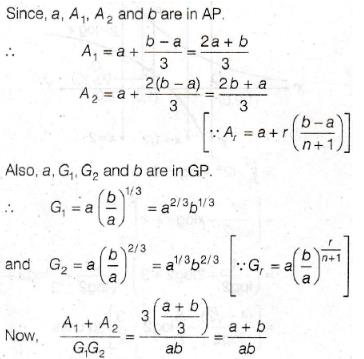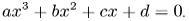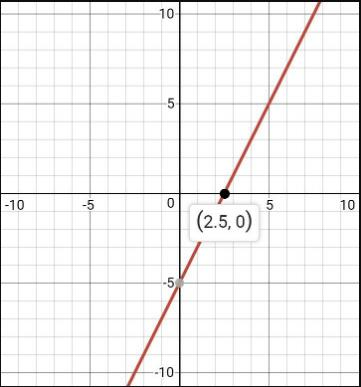Courses

# Test: Geometric Meaning Of The Zeroes

## 10 Questions MCQ Test Mathematics (Maths) Class 10 | Test: Geometric Meaning Of The Zeroes

Description
This mock test of Test: Geometric Meaning Of The Zeroes for Class 10 helps you for every Class 10 entrance exam. This contains 10 Multiple Choice Questions for Class 10 Test: Geometric Meaning Of The Zeroes (mcq) to study with solutions a complete question bank. The solved questions answers in this Test: Geometric Meaning Of The Zeroes quiz give you a good mix of easy questions and tough questions. Class 10 students definitely take this Test: Geometric Meaning Of The Zeroes exercise for a better result in the exam. You can find other Test: Geometric Meaning Of The Zeroes extra questions, long questions & short questions for Class 10 on EduRev as well by searching above.
QUESTION: 1

### Let A1, A2, be two AM’s and G1, G2 be two GM’s between a and b,then (A1 + A2) / G1G2 is equal to :

Solution:QUESTION: 2

### If the point (5,0), (0-2) and (3,6) lie on the graph of a polynomial. Then which of the following is a zero of the polynomial?

Solution:

We obtain zeros of the polynomial when y=0. Observing the points we see that we have point (5,0) which means when y=0 , x= 5 this means x=5 is a solution of the polynomial.

QUESTION: 3

### A polynomial of degree three is called ……

Solution:

cubic polynomial is a polynomial of the form. A cubic polynomial is a polynomial of degree 3. A univariate cubic polynomial has the form . An equation involving a cubic polynomial is called a cubic equation. A closed-form solution known as the cubic formula exists for the solutions of an arbitrary cubic equation.

QUESTION: 4

A fourth degree polynomial is called

Solution:

A polynomial is a mathematical expression which is sum of several terms consisting the same variable in different powers. The degree of the polynomial is the highest power the variable is having in the expression. A quartic polynomial is a polynomial with degree 4. A polynomial with degree 4 can have 0, 1, 2, 3, or 4 roots. There are few methods developed to find the roots of a quartic equation, like Ferrari's solution, changing the equation to depressed form and then solve it or solving a quartic using radicals. These methods are little complex. A quartic function can have 0, 1 or 2 inflection points and have these inflection points implies the concept of golden ratio.

QUESTION: 5

The graph of a quadratic polynomial is a parabola which cuts the X- axis at

Solution:
QUESTION: 6

The graph of the polynomial p(x) intersects the x-axis three times in distinct points, then which of the following could be an expression for p(x):

Solution:

The polynomial intersects the x-axis three times. A point on x-axis is of the form (a,0) where a is any number.So this means that any point on x axis has y coordinate equal to zero and we know that y=0 is when we have roots of the equation.So since there are three points at which y=0 so we have 3 roots of the equation. No. of roots is equal to the degree of the polynomial. So the degree of the polynomial is 3.

QUESTION: 7

The graph of the polynomial f(x) = 2x – 5 crosses the X-axis at the point

Solution:

The graph of the polynomial
f (x) = 2x - 5

Method.

Let us consider, y = f (x) = 2x - 5

Now, when x = 0, y = - 5

and when y = 0, x = 5/2

So, we get two points (0, - 5) and (5/2, 0).

We plot the two points on a graph paper considering two rectangular axes XOX' and YOY'. Then, we find a straight line as shown in the graph.

We see that the line intersects x-axis at the point (5/2, 0).QUESTION: 8

If “1” is a zero of the polynomial P(a) = x2a2 – 2xa + 3x – 2, then x = ______

Solution:

The correct solution of this question is given below:

Here, P(a) = x2a2 - 2xa + 3x - 2

1 is a zero of P(a), so P(1) = 0

Therefore, x212 - 2x.1 + 3x - 2 = 0

x2 + x - 2 = 0

(x + 2)(x - 1) = 0

x = -2, 1

QUESTION: 9

Number of observations are 30 and value of arithmetic mean is 15 then sum of all values is

Solution:
QUESTION: 10

If an+1 + bn+1/an + bis geometric mean between a and b, then n =

Solution: